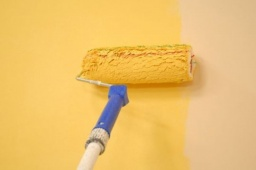# Our house

Our house would paint by four painters in 14 days. How long could it paint five painters? How long will it take if two other painters come to help after five days?

a =  11.2 d
b =  11 d

### Step-by-step explanation:

4·14 = 4·5 + (b-5)·(4+2)

6b = 66

b = 66/6 = 11

b = 11

Our simple equation calculator calculates it.Did you find an error or inaccuracy? Feel free to write us. Thank you!

Tips for related online calculators
Do you have a linear equation or system of equations and looking for its solution? Or do you have a quadratic equation?
Do you want to convert time units like minutes to seconds?